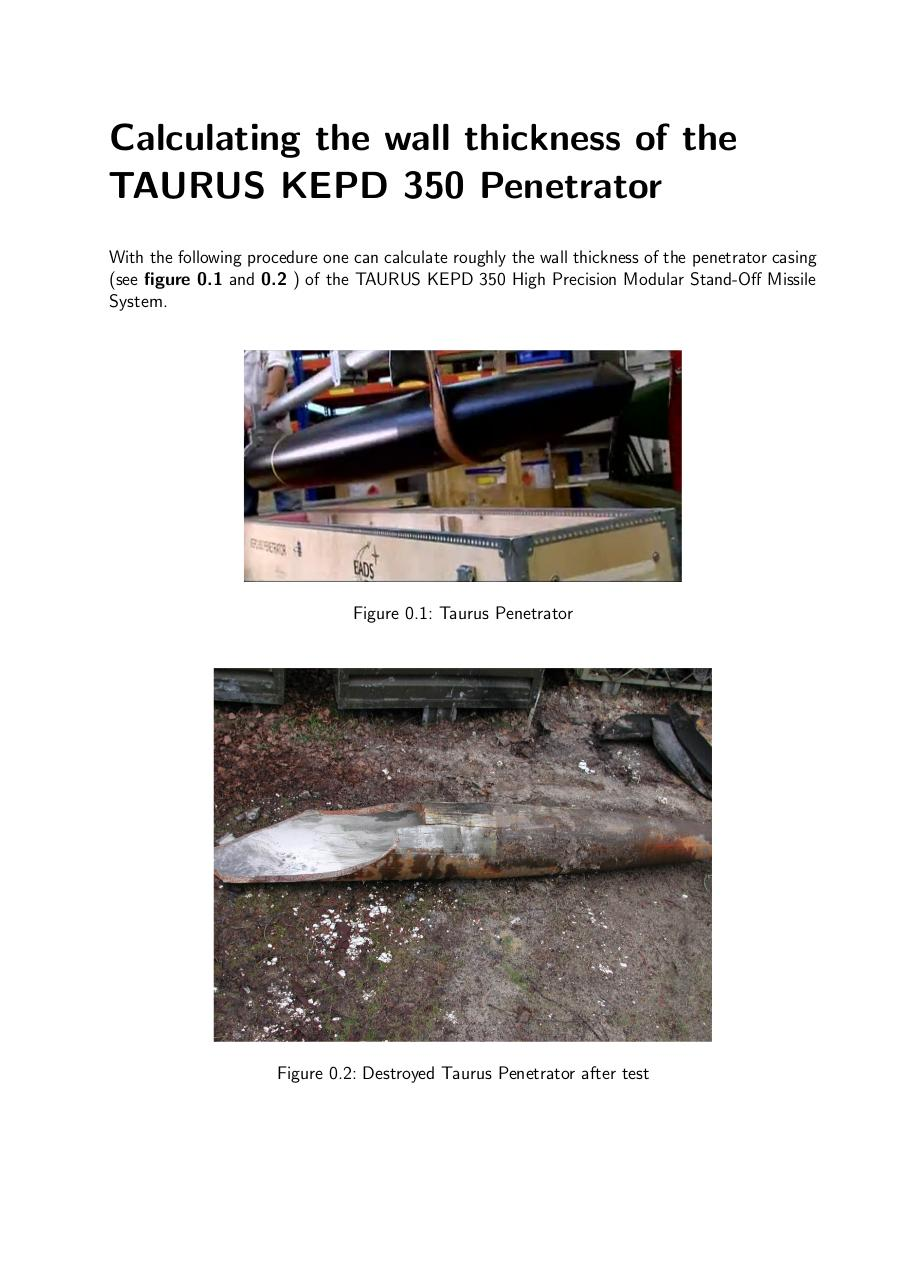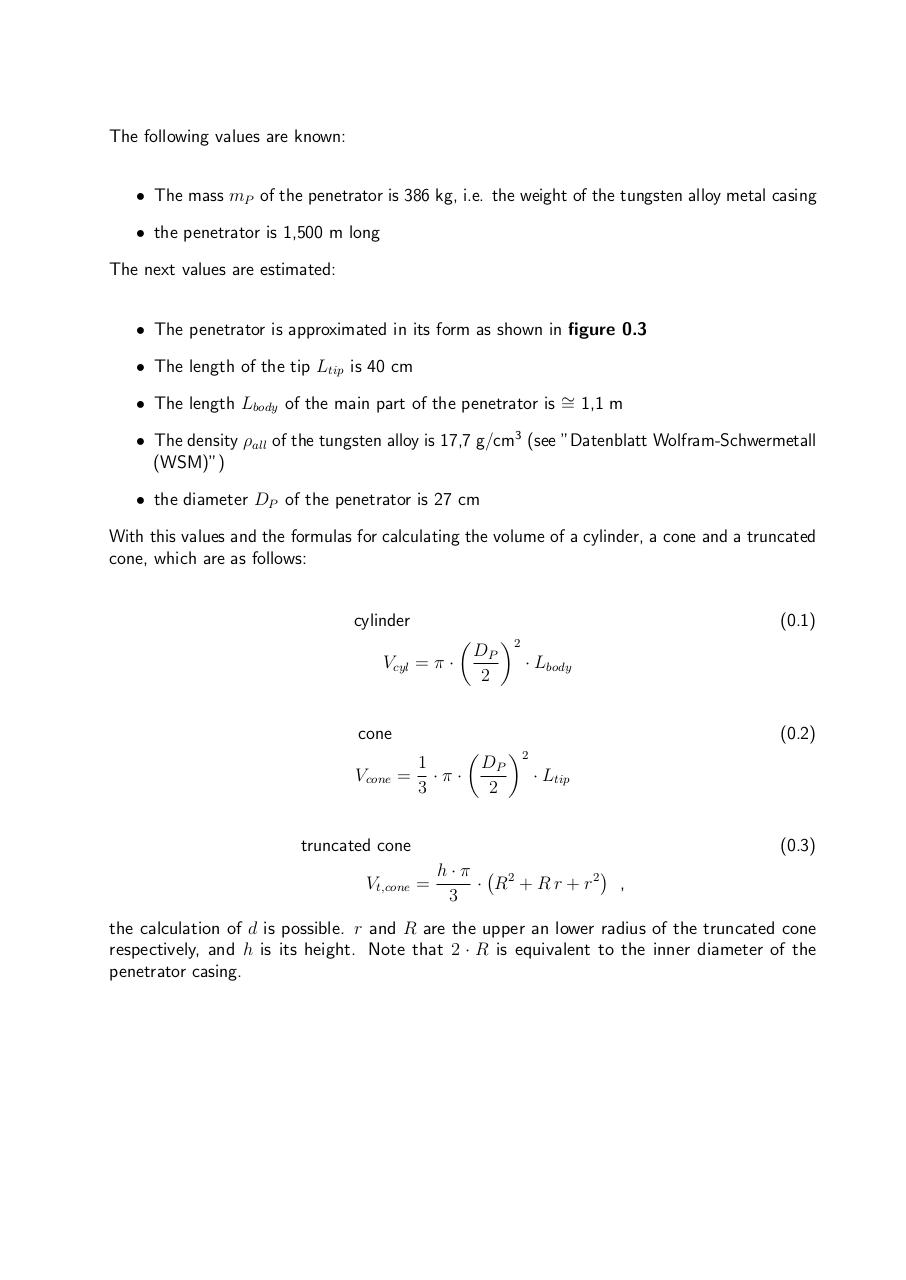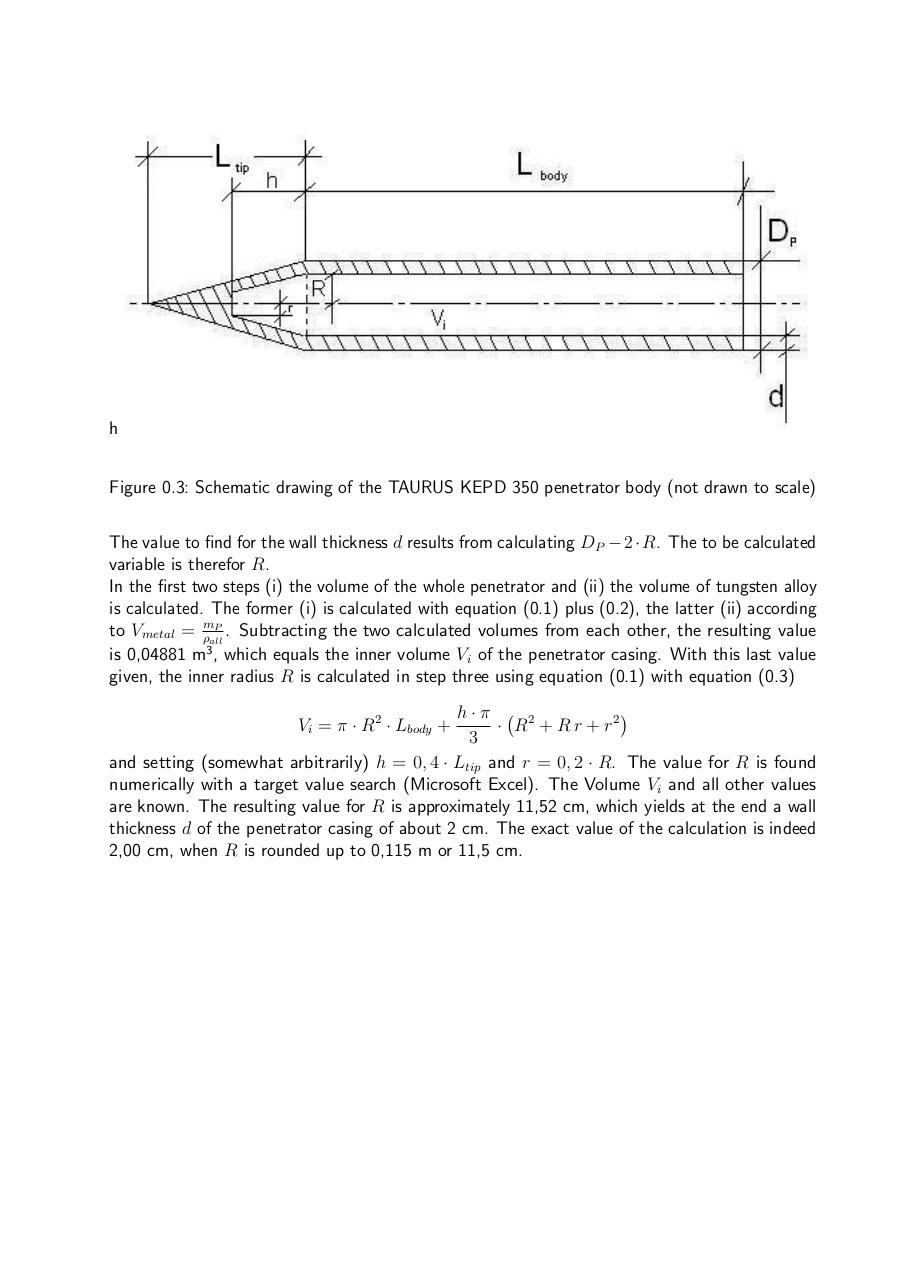# KEPD 350. Penetrator. Thickness shell of Penetrator .pdf

### File information

Original filename: KEPD 350. Penetrator. Thickness shell of Penetrator.pdf

This PDF 1.5 document has been generated by TeX / MiKTeX pdfTeX-1.40.20, and has been sent on pdf-archive.com on 02/12/2019 at 22:11, from IP address 87.143.x.x. The current document download page has been viewed 209 times.
File size: 679 KB (3 pages).
Privacy: public file

KEPD 350. Penetrator. Thickness shell of Penetrator.pdf (PDF, 679 KB)

### Document preview

Calculating the wall thickness of the
TAURUS KEPD 350 Penetrator
With the following procedure one can calculate roughly the wall thickness of the penetrator casing
(see figure 0.1 and 0.2 ) of the TAURUS KEPD 350 High Precision Modular Stand-Off Missile
System.

Figure 0.1: Taurus Penetrator

Figure 0.2: Destroyed Taurus Penetrator after test

The following values are known:
• The mass mP of the penetrator is 386 kg, i.e. the weight of the tungsten alloy metal casing
• the penetrator is 1,500 m long
The next values are estimated:
• The penetrator is approximated in its form as shown in figure 0.3
• The length of the tip Ltip is 40 cm
• The length Lbody of the main part of the penetrator is ∼
= 1,1 m
• The density ρall of the tungsten alloy is 17,7 g/cm3 (see ”Datenblatt Wolfram-Schwermetall
(WSM)”)
• the diameter DP of the penetrator is 27 cm
With this values and the formulas for calculating the volume of a cylinder, a cone and a truncated
cone, which are as follows:

cylinder

(0.1)


Vcyl = π ·

DP
2

2
· Lbody

cone
Vcone =

(0.2)
1
·π·
3



DP
2

2
· Ltip

truncated cone
Vt,cone =

(0.3)

h·π
· R2 + R r + r 2 ,
3

the calculation of d is possible. r and R are the upper an lower radius of the truncated cone
respectively, and h is its height. Note that 2 · R is equivalent to the inner diameter of the
penetrator casing.

h
Figure 0.3: Schematic drawing of the TAURUS KEPD 350 penetrator body (not drawn to scale)
The value to find for the wall thickness d results from calculating DP − 2 · R. The to be calculated
variable is therefor R.
In the first two steps (i) the volume of the whole penetrator and (ii) the volume of tungsten alloy
is calculated. The former (i) is calculated with equation (0.1) plus (0.2), the latter (ii) according
to Vmetal = ρmallP . Subtracting the two calculated volumes from each other, the resulting value
is 0,04881 m3 , which equals the inner volume Vi of the penetrator casing. With this last value
given, the inner radius R is calculated in step three using equation (0.1) with equation (0.3)

h·π
· R2 + R r + r 2
3
and setting (somewhat arbitrarily) h = 0, 4 · Ltip and r = 0, 2 · R. The value for R is found
numerically with a target value search (Microsoft Excel). The Volume Vi and all other values
are known. The resulting value for R is approximately 11,52 cm, which yields at the end a wall
thickness d of the penetrator casing of about 2 cm. The exact value of the calculation is indeed
2,00 cm, when R is rounded up to 0,115 m or 11,5 cm.
Vi = π · R2 · Lbody +#### HTML Code

Copy the following HTML code to share your document on a Website or Blog

#### QR Code### Related keywords